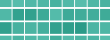# InetSoft Product How-To: Calculating the Page Total

Calculating the page total itself is very simple. First declare two report-level variables in the onLoad Handler, total1 and total2. These will be used to store the totals over 1000 and below 1000 on the current page.

```var total1 = 0; // for total over 1000
var total2 = 0; // for total under 1000 ```

Next, add a script to the total field (Text) in the Content band to add the total value to the two total variables, and to display the appropriate highlighting.

```// calculate page total and highlight
if(value > 1000) {
total1 += value;
band.background = [0,255,0]; // green
}
else {
total2 += value;
band.background = [255,255,255]; // white
}```

Finally, add an onPageBreak Handler script to display the two totals in the footer, and to reset the total variables.

```// assign to footer text element
totalOver.text = formatNumber(total1, "\$#,##0.00");
totalBelow.text = formatNumber(total2, "\$#,##0.00");

// reset totals
total1 = 0;
total2 = 0;```

## Band Rewinding

One complication of page-level calculation is the fact that a band can be rewound to a new page during printing. Therefore, if you leave the report as it currently is, the page total may contain an extra value from the next page. To address this, you will add logic to handle the rewinding of a band.

To “undo” the effect of rewinding, you first need to determine what the last band did before it was rewound. In the onPageBreak Handler handler, you can check if the last band was rewound, and undo the last action. For this example, declare a report-level variable in the onLoad Handler to hold the last value added to the total variables.

`var lastValue = 0; `

In the script for the total field, simply store the last number added to the total in the variable.

`lastValue = value; `

Finally, add code to the onPageBreak script to determine if rewinding occurred, and to undo its effect.

```// if rewound, undo the last value total
if(event.rewound) {
if(lastValue > 1000) {
total1 -= lastValue;
}
else {
total2 -= lastValue;
}
}```

Since you don't want to lose the last value for the next page, add another block at the end of the onPageBreak to push the lastValue to the next page's totals.

```// if rewound, push last value to next page
if(event.rewound) {
if(lastValue > 1000) {
total1 = lastValue;
}
else {
total2 = lastValue;
}
}```Read how InetSoft was rated #3 for implementation in G2 Crowd's user survey-based index.

 Previous: Banded Reports - Rewound Property